# Pairs of Angles – Lines & Angles

• Difficulty Level : Easy
• Last Updated : 15 Dec, 2020

When two lines share a common endpoint, called Vertex then an angle is formed between these two lines is known as the pair of angles. Below is the pictorial representation of the pair of angles.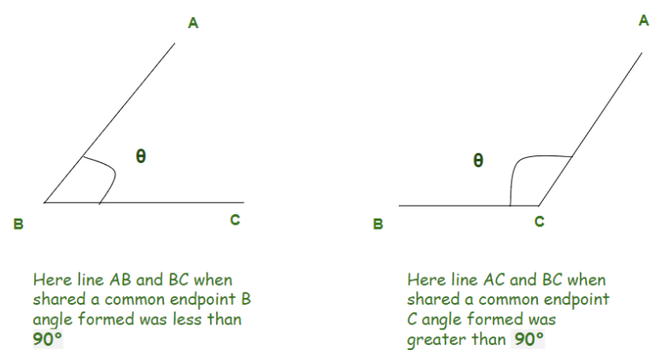Attention reader! All those who say programming isn't for kids, just haven't met the right mentors yet. Join the  Demo Class for First Step to Coding Coursespecifically designed for students of class 8 to 12.

The students will get to learn more about the world of programming in these free classes which will definitely help them in making a wise career choice in the future.

Some of the pair of angles we saw is below:

• Complementary Angles
• Supplementary Angles
• Linear Pair of Angles
• Vertical Angles

### Complementary Angles

When we have two angles whose addition equals 90° then the angles are called Complementary Angles.

Example:

50° and  40° (50° + 40° = 90°)

70° and  20° (70° + 20° = 90°)

Below is the pictorial representation of the Complementary Angles.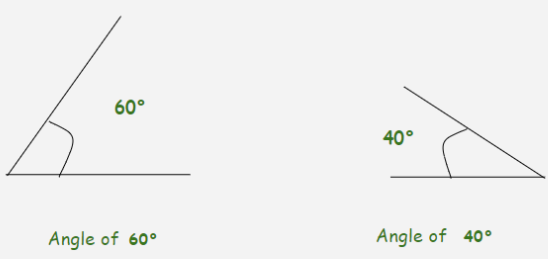• If we have two angles as x° and  y° and  x° +  y° =  90° then x is called the complementary angle of y and y is called the complementary angle of x.

Example: We have  20° and  70° then, 20° is a complementary angle of 70° and  70° is a complementary angle of  20°.

• If we have one angle as  x° then to find a complementary angle we need to subtract it from  90°.

Example: We have  30° then the complementary angle of it is  90° –  30° which is  60°

### Supplementary Angles

When we have two angles whose addition equals to  180° then the angles are called Supplementary Angles.

Example:

150° and  30° (150° + 30° =  180°)

70° and  110° (70° + 110° =  180°)

Below is the pictorial representation of the Supplementary Angle.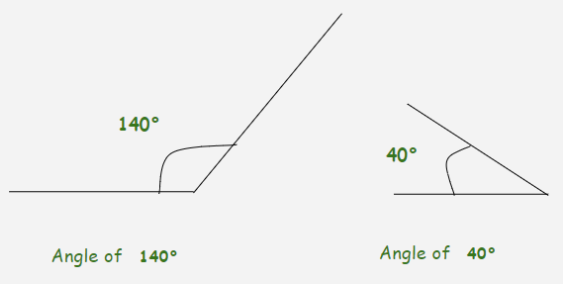• If we have two angles as x° and y° and  x° +  y° = 180° then x is called the supplementary angle of y and y is called the supplementary angle of x.

Example: We have  100° and  80° then, 100° is the supplementary angle of  80° and  80° supplementary angle of  100°.

• If we have one angle as  x° then to find a supplementary angle we need to subtract it from 180°.

Example: We have  60° then the supplementary angle of it is  180° –  60° which is 120°

### Difference Between Complementary Angle and Supplementary Angle

When we have two angles with a common side, a common vertex without any overlap we call them Adjacent Angles.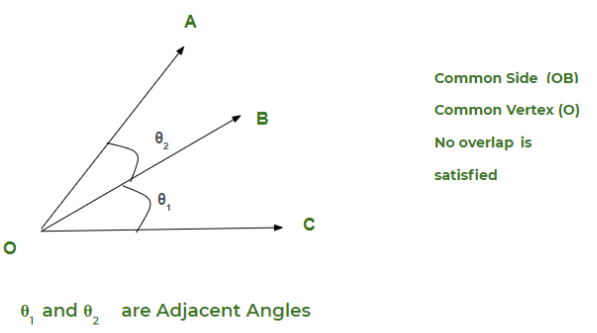We know what conditions two angles need to fulfill to be Adjacent angles. Let’s see some of the examples where we might get confused that whether they are adjacent angles or not.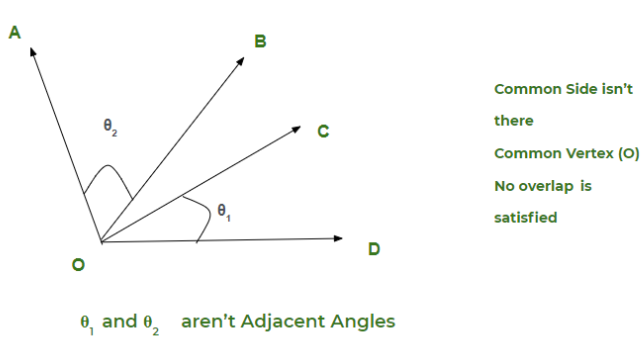Here  θ1 and  θ2 are having a common vertex, they don’t overlap but because they don’t share any common side they aren’t Adjacent Angles.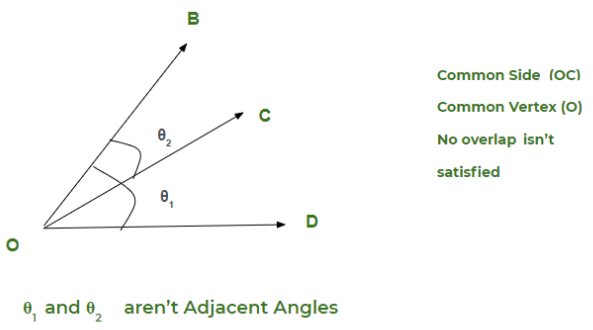Here  θ1 and  θ2 are having a common vertex, they share a common side but they overlap so they aren’t Adjacent Angles.

### Linear Pair of Angles

We say two angles as linear pairs of angles if both the angles are adjacent angles with an additional condition that their non-common side makes a Straight Line.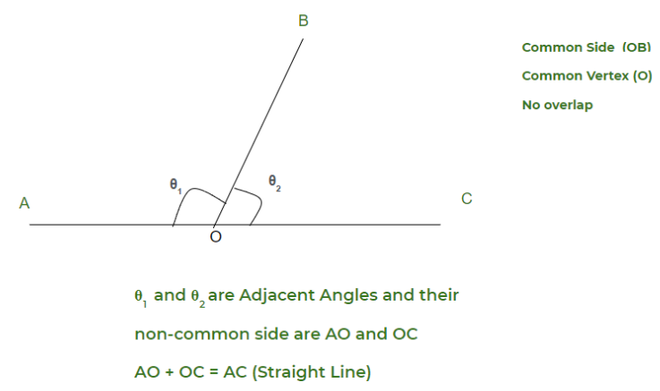Let’s see some examples for a better understanding of Pair of Angles.

Example 1: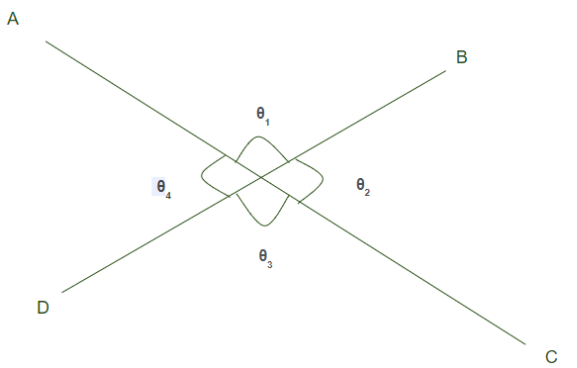Let’s call the intersection of line AC and BD to be O. Now we see four angles are there let’s try to observe them one by one.

• θ1 and θ2 are adjacent angles and their non-common sides are AO and OC, AO + OC = AC is a Straight Line so both are linear pairs of angles.
• θ2 and θ3 are adjacent angles and their non-common sides are BO and OD, BO + OD = BD is a Straight Line so both are linear pairs of angles.
• θ3  and θ are adjacent angles and their non-common sides are CO and OA, CO + OA = CA is a Straight Line so both are linear pairs of angles.
• θ4  and θ1  are adjacent angles and their non-common sides are D0 and OB, DO + OB = DB is a Straight Line so both are linear pair of angles.

### Vertical Angles

A vertical angle is a pair of non-adjacent angles that are formed by the intersection of two Straight Lines.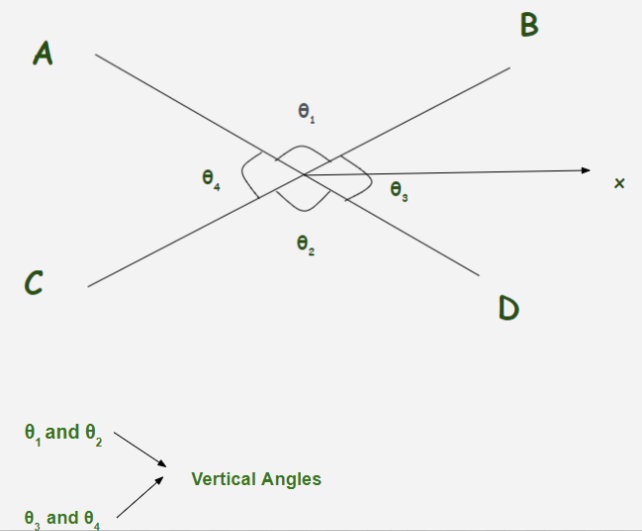Here we see line  AD and line BC intersect at one point  let’s call it X and thus four angles are formed

∠AXB =  θ1

∠BXD =  θ2

∠DXC =  θ3

∠CXA =  θ4

θ and θ2 are non-adjacent angles and formed by the intersection of line  AD and BC therefore they are Vertical Angles are always Equal so θ1 = θ2. Similarly, θ3 and θ4 are also vertical angles therefore θ3 = θ4. Let’s try to understand with a question: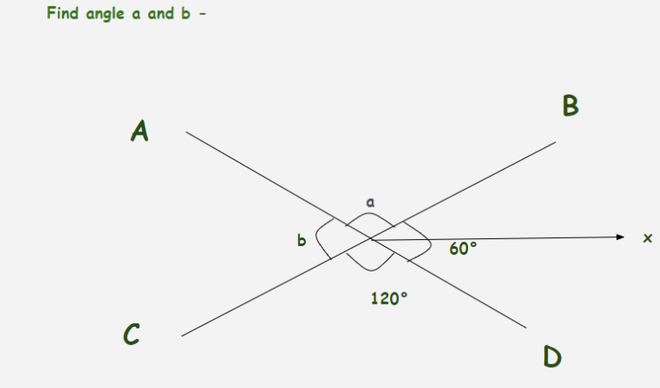Here we see ∠BXD  and b are vertically opposite angles therefore

b = ∠BXD

b = 60°

and we also see that ∠DXC and a are vertically opposite angles therefore

a = ∠DXC

a = 120°

My Personal Notes arrow_drop_up